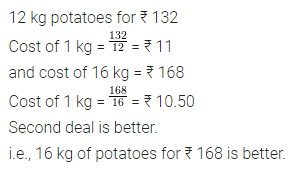ML Aggarwal Class 7 Solutions Chapter 6 Ratio and Proportion Ex 6.3 for ICSE Understanding Mathematics acts as the best resource during your learning and helps you score well in your exams.

## ML Aggarwal Class 7 Solutions for ICSE Maths Chapter 6 Ratio and Proportion Ex 6.3

Question 1.
6 bowls cost ₹ 90. What would be the cost of 10 such bowls?
Solution: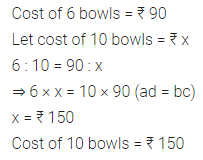Question 2.
Ten pencils cost ₹ 15. How many pencils can be bought with ₹ 72?
Solution: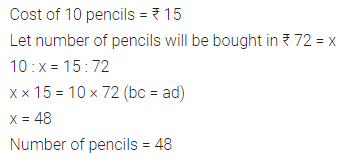Question 3.
400 grams cake costs 80 rupees. How much would a 1.5 kg cake cost?
Solution:Question 4.
A man earns ₹ 18000 in 3 months.
(i) How much time would he take to earn ₹ 30000?
(ii) How much money will he earn in 7 months?
Solution:Question 5.
12 mangoes weigh 2.4 kg. What is the weight of 8 mangoes?
Solution: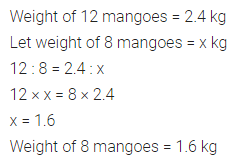Question 6.
If the weight of 12 sheets of thick paper is 40 grams, how many sheets of the same paper would weigh 2$$\frac { 1 }{ 2 }$$ kilograms?
Solution: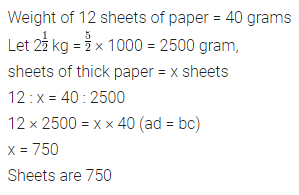Question 7.
A bus consumes 25 litres of diesel in covering a distance of 90 kilometres. How much diesel is needed to cover 288 kilometres?
Solution:Question 8.
If $$\frac { 4 }{ 5 }$$ metre cloth costs ₹ 36, find the cost of 2$$\frac { 1 }{ 5 }$$ metres of cloth.
Solution: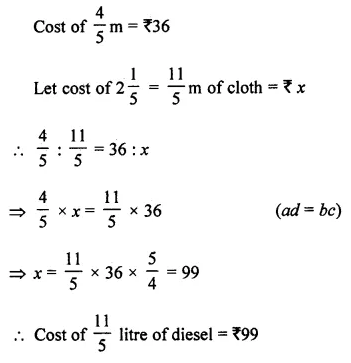Question 9.
If 15 men can pack 540 parcels per day, how many men are needed to pack 396 parcels per day?
Solution: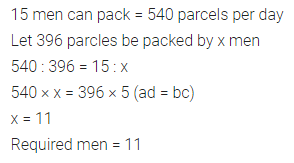Question 10.
Which is a better buy 12 kg potatoes for ₹ 132 or 16 kg potatoes for ₹ 168?
Solution: